# An Improved Long Short-Term Memory Neural Network for Macroeconomic Forecast

An Improved Long Short-Term Memory Neural Network for Macroeconomic Forecast
Department of Management, Shijiazhuang Vocational Technology Institute, Shijiazhuang 050081, China

Corresponding Author Email:
shiliu20161006@126.com
Page:
577-584
|
DOI:
https://doi.org/10.18280/ria.340507
12 April 2020
|
Accepted:
28 August 2020
|
Published:
20 November 2020
| Citation

OPEN ACCESS

Abstract:

The statistics and cyclical swings of macroeconomics are necessary for exploring the internal laws and features of the market economy. To realize intelligent and efficient macroeconomic forecast, this paper puts forward a macroeconomic forecast model based on improved long short-term memory (LSTM) neural network. Firstly, a scientific evaluation index system (EIS) was constructed for macroeconomy. The correlation between indices was measured by Spearman correlation coefficient, and the index data were preprocessed by interpolating the missing items and converting low-frequency series into high-frequency series. Next, the corresponding mixed frequency dataset was constructed, followed by the derivation of the state space equation. Then, the LSTM neutral network was optimized by the Kalman filter or macroeconomic forecast. The effectiveness of the proposed forecast method was verified through experiments. The research results lay a theoretical basis for the application of LSTM in financial forecasts.

Keywords:

long short-term memory (LSTM), neural network, macroeconomics, economic forecast, mixed frequency

1. Introduction

In recent years, China has witnessed rapid economic growth and continued improvement of market economic system. To ensure the rapid, stable, and healthy development of the economy, the policymakers need to understand the laws and features of the market economy, and make major economic decisions in line with these laws [1-3].

The core issues in macroeconomics are statistics and cyclical swings [4-7]. The macro-control system of the government relies on the accurate measurement and forecast of macroeconomics. The measured and forecast results provide the basis for the prediction of economic trends, and an important reference for the positioning of future macroeconomic policies.

The existing studies on macroeconomic forecast mainly tackle three issues: the monitoring and forecast of economic swings, the phased identification of macroeconomic situation and turning points, and the forecast of macroeconomic growth rate [7-9].

First, economic swings are mostly monitored and forecasted with economic prosperity index or structural measurement model. The former is a nonparametric method without a specific model, while the former is a parametric method based on a specific model [10, 11]. Ramahatana and David  theoretically differentiated between the quarterly swings, long-term trends, cyclic swings, and irregular swings in economic time series, and evaluated the correlations between the rigid factors of cyclic economic swings by analyzing the intertemporal correlations among economic time series. Khalid  innovatively defined the growth cycle swings of macroeconomy, and removed the dominant indices on economic trend from the monitoring and forecast of economic swings, leaving only the indices affected by cyclic elements.

Second, the phased identification of macroeconomic situation and turning points could be realized through parametric or nonparametric methods [14-16]. Apergis  proposed the Bry Boschan Quarterly (BBQ) algorithm to determine the turning points in quarterly economic time series, and presented two constraints for the determination: (1) the peak-to-valley interval or valley-to-peak interval is at least half a year; (2) a swing cycle, i.e. the interval between two identical turning points is at least four quarters. Perez et al.  constructed a multivariate model by expanding the Markov regime switching time series model in variable dimension and applicable scope. And they proposed the expectation-maximization (EM) algorithm, which excels in handling evaluation indices, as well as fragmented, missing, and unbalanced data, revised the relationship between the forecast results and the newly released economic indices, and measured the contribution of each index to the forecast. Bexten et al.  established a Markov regime switching - vector autoregressive model that can handle quarterly gross domestic product (GDP) data of different frequencies, and effectively identified the turning points in the macroeconomy of the United States (US).

Third, macroeconomic growth rate is generally forecasted based on models or the synthetic index released by National Bureau of Economic Research (NBER) [20-22]. Udagawa et al.  combined static Probit model and Monte-Carlo (MC) simulation to analyze the long- and short-term spreads, and forecasted the turning point of US economic recession. Shapot, and Shapot  presented an unconstrained vector autoregressive model, which overcomes the large forecast errors of large macroeconomic models induced by the lack of empirical evidences and theoretical supports.

With strong self-learning ability and self-adaptability, artificial neural network (ANN) has obvious advantages over traditional nonlinear economic forecast methods. To realize intelligent and efficient macroeconomic forecast, this paper puts forward a macroeconomic forecast model based on improved long short-term memory (LSTM) neural network. Firstly, an evaluation index system (EIS) was built up for macroeconomy, and the correlation between indices was measured by Spearman correlation coefficient. In addition, the index data were preprocessed by interpolating the missing items and converting low-frequency series into high-frequency series. Based on the processed data, the authors established the corresponding mixed frequency dataset and state space model. After that, the LSTM neutral network was optimized by the Kalman filter or macroeconomic forecast, and proved effective through experiments.

2. EIS Construction and Data Preprocessing

Referring to China Macroeconomic Indicators, the scale and quality of China’s macroeconomy can be comprehensively measured in terms of industry, fixed asset investment, domestic commerce, total import and export, utilization of foreign capital, public finance, finance, securities, and prices.

Figure 1 presents the curve of China’s macroeconomic leading index. Considering these metrics of macroeconomy, a scientific hierarchical EIS for macroeconomy was established in the principles of comprehensive, comparability and operability. The proposed EIS contains 9 primary indices and 31 secondary indices.

## 1.png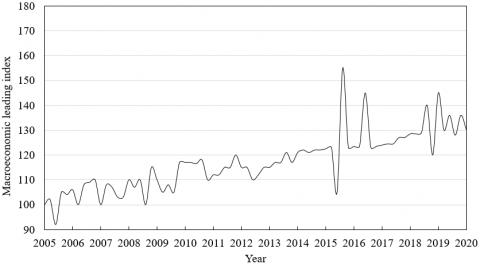Figure 1. The curve of China’s macroeconomic leading index

Layer 1 (goal):

C={macrocosmic evaluation}

Layer 2 (primary indices):

C={C1, C2, C3, C4, C5, C6, C7, C8, C9}=industrial output, fixed asset investment, domestic commerce, total import and export, utilization of foreign capital, public finance, finance, securities, prices};

Layer 3 (secondary indices):

C1={C11, C12, C13, C14, C15, C16, C17}={value added of industrial enterprises above designated size, extractive industries, manufacturing, electricity, gas and water production and supply, state holding enterprises, joint-stock cooperative enterprises, enterprises funded by foreign investors or investors from Hong Kong, Macao, and Taiwan};

C2={C21, C22, C23}={fixed asset investment, state-owned investment, investment in real estate development};

C3={C31, C32, C33, C34, C35}={total retail sales of consumer goods, urban employment rate, rural employment rate, retail income, food service income};

C4={C41, C42}={export value, import value};

C5={C51}={actual foreign direct investment};

C6={C61, C62, C63}={fiscal revenue (excluding debt revenue), various taxes, fiscal expenditure (excluding debt expenditure)}

C7={C71, C72, C73, C74, C75, C76}={currency and quasi-currency (M2), currency (M1), cash flow (M0), deposit balance of financial institutions, household deposit, loan balance of financial institutions};

C8={C81, C82}={Shanghai Stock Exchange Composite Index, Shenzhen Stock Exchange Component Index}

C9={C91, C92, C93, C94}={general consumer price index, retail price index of domestic products, retail price index of export products, retail price index of import products}.

Considering the types of evaluation indices, the Spearman correlation coefficient, which reflects the dependence between the two evaluation datasets, was chosen to measure the correlation between the degree of linear correlation between the evaluation indices.

Let Ci and Cj be two primary index datasets, both of which contain m index data. First, the index data in the two datasets were sorted in descending order separately, and replaced with the data with the corresponding rankings. The Spearman correlation coefficient between Ci and Cj can be calculated by:

$s=\frac{\sum{\left( {{U}_{i}}-\bar{U} \right)\left( {{V}_{i}}-\bar{V} \right)}}{\sqrt{\sum{{{\left( {{U}_{i}}-\bar{U} \right)}^{2}}\sum{{{\left( {{V}_{i}}-\bar{V} \right)}^{2}}}}}}$        (1)

where, Ui and Vi are the rankings of primary index data; U and $\bar{V}$ are the means of the corresponding ranking data. In actual application, the Spearman correlation coefficient can be calculated based on the difference ei of each pair of index data in Ci and Cj in the corresponding ranking data:

$s=1-\frac{\eta \sum{e_{i}^{2}}}{m({{m}^{2}}-1)}$       (2)

## 2.png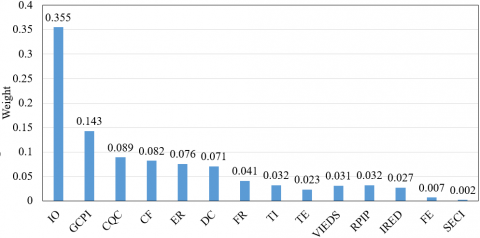Figure 2. The weight distribution of the index combination

Note: IO is Industrial output; GCPI is General consumer price index; CQC is Currency and quasi-currency; CF is Cash flow; ER is Employment rate; DC is Domestic commerce; FR is Fiscal revenue; TI is Total import; TE is Total export; VIEDS is Value added of industrial enterprises above designated size; RPIP is Retail price indices of products; IRED is Investment in real estate development; FE is Fiscal expenditure; SECI is Stock exchange composite indices.

The Spearman correlation coefficient obtained by formula (2) characterizes the directions of primary index datasets Ci and Cj. Suppose Ci is an independent macroeconomic evaluation index, and Cj is a dependent macroeconomic evaluation index. If the secondary index data under Ci increase, and if those under Cj also increase, then the Spearman correlation coefficient between the two datasets is positive; otherwise, the coefficient is negative. If the coefficient is 1, then the two datasets have a completely monotonous correlation; if the coefficient is 0, then the two datasets have no correlation. Figure 2 shows the weight distribution of the index combination obtained through correlation analysis.

The next is to analyze the Granger causality between the two primary index datasets Ci and Cj. During the prediction of Cj, Ci has an impact on the change law of Cj, if Cj can be predicted more effectively based on the historical information of Ci and Cj than based on the historical information of Cj alone. In this case, Ci is the Granger cause of Cj. The flow of the Granger causality test under this condition is explained in Figure 3.

## 3.png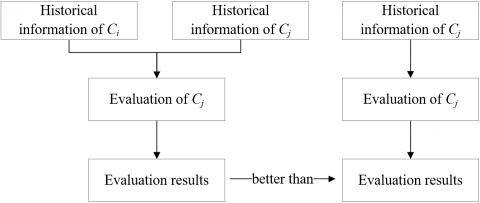Figure 3. The flow of Granger causality test

The missing items in macroeconomic index data are usually interpolated or deleted. Here, the missing items in secondary index data are complemented through cubic spline interpolation. Let {Cij1,Cij2,…,Cijp} be the data of secondary index dataset Cij. Depending on the actual situation, an independent variable time series A={a1,a2,…,ap} was added to dataset Cij. Taking data in A as boundaries, dataset Cij was split into p-1 intervals. Then, the cubic spline interpolation function must meet the following conditions:

(1) The function is continuous at the boundary of adjacent intervals, passing through every data in the time series:

$Splin{{e}_{k}}({{a}_{k}})={{C}_{ijk}}$       (3)

$Splin{{e}_{k}}({{a}_{k+1}})={{C}_{ijk+1}}$        (4)

(2) The curves of the first- and second-order partial derivatives of the function are smooth and continuous in each interval [ak, ak+1]:

$Splin{{{e}'}_{k}}({{a}_{k+1}})=Splin{{{e}'}_{k+1}}({{a}_{k+1}})$      (5)

$Splin{{{e}''}_{k}}({{a}_{k+1}})=Splin{{{e}''}_{k+1}}({{a}_{k+1}})$       (6)

The cubic polynomial corresponding to each interval can be expressed as:

\begin{align} & Splin{{e}_{k}}(a)={{b}_{1k}}{{\left( a-{{a}_{i}} \right)}^{3}}+{{b}_{2k}}{{\left( a-{{a}_{i}} \right)}^{2}} \\ & \text{ }+{{b}_{3k}}\left( a-{{a}_{i}} \right)+{{b}_{4k}} \\\end{align}        (7)

where, b1k, b2k, b3k, and b4k contain a total of 4 (p-1) unknown polynomial coefficients.

(3) The function needs to meet the natural boundary conditions, i.e., the second-order derivatives are zero at the left and right ends of the time series:

$Splin{e}''({{a}_{1}})=Splin{e}''({{a}_{m}})=0$       (8)

The function also needs to meet fixed boundary conditions, i.e. the second-order derivatives c1 and c2 at the left and right ends of the time series are fixed constant values:

$Splin{e}''({{a}_{1}})={{c}_{1}}$       (9)

$Splin{e}''({{a}_{m}})={{c}_{2}}$      (10)

By selecting a boundary condition and solving the 4 (p-1) equations, all unknown polynomial coefficients could be obtained, and the cubic spline interpolation function could be determined for each interval. Then, the interpolation results can be obtained by substituting the independent variable value that corresponds to each missing item of the index data was substituted to the corresponding expression.

In this paper, the LSTM neural network is improved to forecast the macroeconomy. The network was trained entirely by time series data. Considering the time scale difference between time series data of the evaluation indices, this paper adopts the Denton method to convert the low-frequency index data to high-frequency format. Let H be the high-frequency series converted from a low-frequency series Lij={Lij1,Lij2,…,Lijp} of secondary indices for macroeconomy, and H' be another high-frequency series with a similar growth rate and the same number n of index data as H. The Denton method needs to satisfy the following constraint:

${{L}_{ijk}}=\sum\limits_{l={{s}_{k}}}^{{{e}_{k}}}{\varepsilon {{H}_{l}}t}$      (11)

where, t is the time point of low-frequency series; sk and ek are the starting and end points of a time interval in high- and low-frequency time series, respectively. The value of constant c must be selected based on the type of data Hl in high-frequency time series H. The minimum penalty function can be expressed by:

$\underset{H}{\mathop{\min PF}}\,\left( H,{H}' \right)={{\sum\limits_{l=2}^{n}{\left( \frac{{{H}_{l}}}{{{{{H}'}}_{l}}}-\frac{{{H}_{l-1}}}{{{{{H}'}}_{l-1}}} \right)}}^{\text{2}}}$      (12)

3. Mixed Frequency Dataset and State Space Model

Figure 4 presents the sketch map of the macroeconomic forecast model. Since the data of different indices vary with statical frequency, this paper prepares a mixed frequency dataset based on the monthly and quarterly data on macroeconomy after frequency transform.

Let {Oqua, t}be the observation series of quarterly indices; {Omon, t} be the observation series of monthly indices; {Rqua, t}be the potential monthly random series of quarterly indices. Then, the quarterly index data can be obtained by superposing the monthly index data:

${{O}_{qua,t}}={{R}_{qua,t}}+{{R}_{qua,t-1}}+{{R}_{qua,t-2}}$      (13)

Formula (13) can be optimized by replacing the arithmetic mean with the geometric mean:

${{O}_{qua,t}}=\text{3}\sqrt[\text{3}]{{{R}_{qua,t}}\cdot {{R}_{qua,t-1}}\cdot {{R}_{qua,t-2}}}$      (14)

## 4.png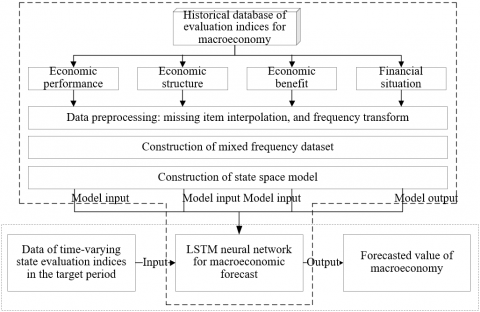Figure 4. The macroeconomic forecast model

Taking the logarithm of formula (14):

$In{{O}_{qua,t}}=In3+\frac{1}{3}\left( In{{R}_{qua,t}}+In{{R}_{qua,t\text{-}1}}+In{{R}_{qua,t\text{-}2}} \right)$      (15)

Then, a 12-order difference year-on-year data model can be constructed as:

$In{{O}_{qua,t}}-In{{O}_{qua,t\text{-}12}}=\frac{1}{3}\left( In{{R}_{qua,t}}-In{{R}_{qua,t\text{-}12}}+In{{R}_{qua,t\text{-}1}}-In{{R}_{qua,t\text{-}13}}+In{{R}_{qua,t\text{-}2}}-In{{R}_{qua,t\text{-}14}} \right)$ (16)

The year-on-year growth rate of quarterly indices can be approximated as ΔOqua, t=InOqua, t -InOqua, t-12, and its potential monthly year-on-year growth rate as ΔRqua, t=InRqua, t -InRqua, t-12. Then, formula (16) can be rewritten as:

${{O}^{*}}_{qua,t}=\frac{1}{3}\Delta {{R}_{qua,t}}+\frac{1}{3}\Delta {{R}_{qua,t-1}}+\frac{1}{3}\Delta {{R}_{qua,t-2}}$       (17)

From the above analysis, compared with quarterly indices, {ΔRqua,t} is the year-on-year growth rate series of unobservable potential monthly indices; {O*qua,t} is the year-on-year growth rate series of observable quarterly indices.

For accurate forecast and evaluation of China’s macroeconomic conditions, it is necessary to fully utilize the high-frequency monthly index data. Hence, a state space model was created based on the mixed frequency dataset. Suppose N endogenous index data contain Nmon monthly indices and Nqua quarterly indices. The index series {Rt} of potential states can be expressed as a d-dimensional autoregressive vector:

${{R}_{t}}={{\tau }_{1}}{{R}_{t-1}}+...+{{\tau }_{d}}{{R}_{t-d}}+\tau$      (18)

where, Rt=[Rmon,tT,Rqua,tT]T; Rmon,t is an Nmon×1-dimensional matrix of the potential monthly year-on-year growth rate series of quarter t; Rqua,t is an Nqua×1-dimensional matrix of the potential monthly year-on-year growth rate series of month t. Then, the state equation of the state space model can be expressed as:

${{D}_{t}}={{K}_{1}}(\tau ){{D}_{t-1}}+K(\tau )$      (19)

where, Dt=[RtT,...,Rt-d+1T]T; τ=[τ1,…,τd,τ]’. The first N rows of K1(τ), and K(τ) were used to generate the d-dimensional autoregressive vector (18), and the other rows were used to generate Rqua,t. Whereas the index data of different months are collected at different time:

${{O}_{mon,t}}={{W}_{mon,t}}{{R}_{mon,t}}={{W}_{mon,t}}{{\Psi }_{monD}}{{D}_{t}}$      (20)

where, Wmon,t is the weight coefficient matrix of the monthly index data obtained in quarter t. Then, Rmon,t was represented by ΨmonDDt, where ΨmonD is the weight coefficient matrix of the state equation. The Oqua,t was observed once every three months. If t is the last month of each quarter, Wqua,t is a unit matrix, and the matrices of the other months are empty:

\begin{align} & {{O}_{qua,t}}={{W}_{qua,t}}{{O}^{*}}_{qua,t} \\ & \text{ }={{W}_{qua,t}}\left( \frac{1}{3}\Delta {{R}_{qua,t}}+\frac{1}{3}\Delta {{R}_{qua,t-1}}+\frac{1}{3}\Delta {{R}_{qua,t-2}} \right) \\ & \text{ }={{W}_{qua,t}}{{\Psi }_{quaD}}{{D}_{t}} \\\end{align}      (21)

Through the above analysis, the measurement equation of the state space model can be expressed as:

${{O}_{t}}={{W}_{t}}{{\Psi }_{D}}{{D}_{t}},$      (22)

4. Improved LSTM for Macroeconomic Forecast

The LSTM is a special recurrent neural network (RNN) to process inputs of data series. In the traditional LSTM, the hidden layer cannot handle relatively long time series. To solve the problem, a unit state module was added to the LSTM for macroeconomic forecast to store the long-term states of the index data time series, such that the laws of the initial elements in the time series can be passed to the latter elements.

The proposed LSTM neural network was developed under the Keras deep learning framework with a TensorFlow backend. Keras is a python deep learning library with good ability of encapsulation. In addition to modeling, training, and learning, Keras can cooperates with the backend engine in implementing the underlying operations of deep learning (e.g. tensor calculations).

## 5.png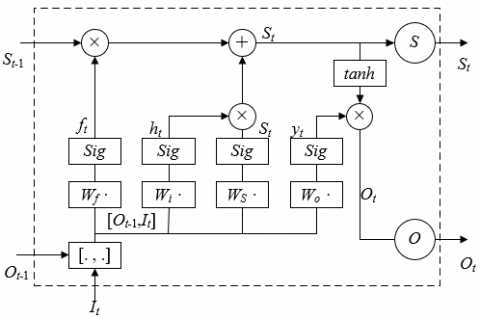Figure 5. The internal structure of LSTM neural network

The internal structure of LSTM neural network is shown in Figure 5. At time t, the LSTM neuron receives the input It of the neural network at time t, the output Ot−1 of the neuron at time t−1, and the unit state St−1 of the memory series at time t−1, and emits the output Ot of the neuron at time t, and the unit state St of the updated series at time t.

The neuron state is controlled by three gates: input gate, forget gate, and output gate. The input gate receives state input and judges how much information needs to be passed to the long-term state; the forget gate judges whether to discard the long-term state; the output gate judges whether to output the updated long-term state. The calculation rule of all the gates can be described as:

$f\left( x \right)=sigmoid\left( W\cdot I+\varepsilon \right)$     (23)

where, W is the weight coefficient of a gate; I is the input data series; b is the bias. If the output of a gate is 0, then the gate is closed; If the output is 1, then the gate is open. Let Wf be the weight matrix of the forget gate during forward calculation. Then, the forget vector can be computed by:

${{f}_{t}}=sigmoid\left( {{W}_{f}}\cdot \left[ {{O}_{t-1}},{{I}_{t}} \right]+{{\varepsilon }_{f}} \right)$      (24)

where, [Ot−1, It] is the input-output vector of the neuron; ԑf is the bias of the forget gate. Let Wi be the weight matrix of the input gate. Then, the input vector can be computed by:

${{h}_{t}}=sigmoid\left( {{W}_{i}}\cdot \left[ {{O}_{t-1}},{{I}_{t}} \right]+{{\varepsilon }_{i}} \right)$      (25)

where, ԑi is the bias of the input gate. Let WS be the weight matrix of the unit state. Then, the unit state vector can be computed by:

$S_{t}^{*}=\tanh \left( {{W}_{S}}\cdot \left[ {{O}_{t-1}},{{I}_{t}} \right]+{{\varepsilon }_{S}} \right)$      (26)

${{S}_{t}}={{f}_{t}}{}^\circ {{S}_{t-1}}+{{h}_{t}}{}^\circ S_{t}^{*}$        (27)

where, S*t is the input unit state of Ot−1 and It passing through the input gate; ԑS is the bias of unit state; ◦ is the multiplication between all vectors by the sequence of the corresponding elements. Let Wo be the weight matrix of the output gate. Then, the output vector can be computed by:

${{y}_{t}}=sigmoid\left( {{W}_{o}}\cdot \left[ {{O}_{t-1}},{{I}_{t}} \right]+{{\varepsilon }_{o}} \right)$      (28)

where, ԑo is the bias of the output gate. Through the above analysis, the final output of the neuron in the LSTM neural network can be obtained by:

${{O}_{t}}={{y}_{t}}{}^\circ \tanh \left( {{S}_{t}} \right)$      (29)

The error of the neuron, i.e., the mean squared error (MSE) can be obtained by:

$MSE=\frac{1}{N}{{\sum{\left( {{Y}^{*}}-Y \right)}}^{2}}$      (30)

where, y* and y are the forecasted result of LSTM neural network, and the actual evaluation result, respectively.

Based on the state space equation and LSTM neural network, the macroeconomic forecast model was optimized by weighted fusion Kalman filter, aiming to obtain more accurate evaluation result.

The state equation constructed in the previous section includes time-varying state evaluation index Dt, a matrix K of the linear relationship between indices, and process noise φt. Without considering the error, it is easy to forecast Ot+1 based on Dt. In fact, the forecasted Ot deviates from the actual Ot+1 by an error Δe, which can be used to correct the forecasted Ot. Since the predicted Ot encompasses KOt and gain ΔK, the state equation of the model can be corrected by:

${{D}_{t}}={{K}_{t-1}}{{D}_{t-1}}+{{K}_{t-1}}+\Delta K$      (31)

To sum up, the LSTM neural network was combined with Kalman filter to forecast the macroeconomy. The Kalman filter mainly eliminates the outliers from the macroeconomic data, while the LSTM neural network is trained by the fitted time series data. In this way, the one-step forecast of macroeconomic indices was converted into a supervised learning problem by ANN.

5. Experiments and Results Analysis

This paper performs a Spearman’s rank correlation test on the correlations between the selected evaluation indices for macroeconomy. Figure 6 presents the linear correlations between the indices. It can be seen that the correlations between the indices are demand-oriented. Starting from the supply side, the commodity market is considered in the dimensions of investment, consumption, and net export demand; the money market is considered in the dimensions of supplies of money and credit, supply, and interest rates. In addition, special consideration is given to resident income, employment rate, state-owned investment, and taxes. Currently, China’s economy is highly resilient, and highly independent of the international economic environment. Therefore, the proposed model provides a benchmark for macroeconomic research.

## 6.pngFigure 6. The linear correlations between evaluation indices

## 7a.png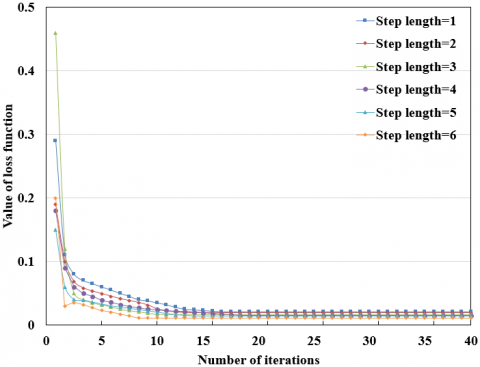(a) 2018

## 7b.png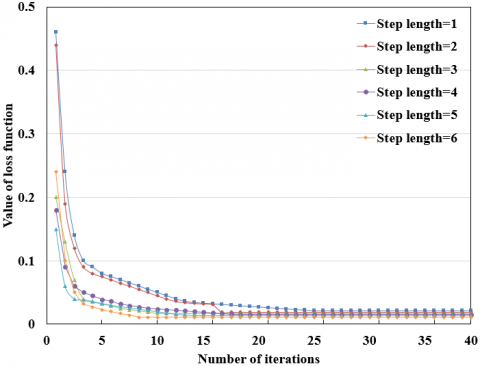(b) 2019

Figure 7. The macroeconomic evaluation results at different step lengths

## 8a.png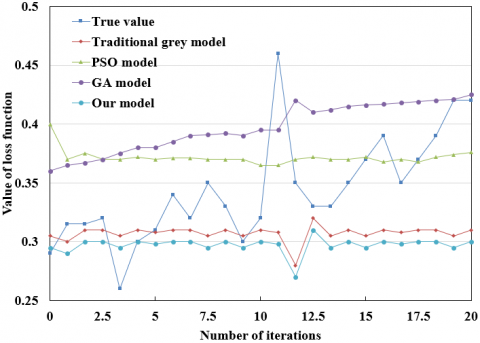(a) 2018

## 8b.png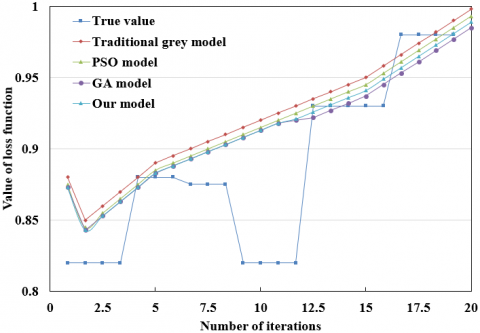(b) 2019

Figure 8. The macroeconomic forecasts of different models

(Note: PSO and GA are short for particle swarm optimization and genetic algorithm, respectively.)

The macroeconomies in 2018 and 2019 were forecasted by the LSTM neural network at different step lengths. As shown in Figure 7, under different step lengths, the training error of the LSTM on different evaluation indices did not fluctuate significantly, but the number of recurrent layers in the network and the length of iterative cycle both increased with the step length.

To verify the effectiveness and accuracy of our method in macroeconomic forecast, the authors attempted to recover the macroeconomic situation from the forecasted results. Figure 8 sort outs the true values and forecasted values by our method, the traditional grey model, the PSO model, and the GA model, for the 24 months in 2018 and 2019. It can be seen that our method achieved much higher forecast accuracy than the other methods.

Table 1 sums up the absolute errors, mean errors, optimal rates, and standard deviations of the four methods in macroeconomic forecast from 2008 to 2019. It can be seen that our method had an obvious advantage over the other methods in absolute error, mean error, optimal rate, and standard deviation. The results show that our method can achieve the highest forecast accuracy and output the most stable results.

Table 1. The absolute errors of macroeconomic forecasts by different methods

 Year Traditional grey model PSO model GA model Our model 2008 0.0154 0.0128 0.0134 0.0107 2009 0.0289 0.0243 0.0232 0.0211 2010 0.0204 0.0187 0.0149 0.0121 2011 0.0242 0.0197 0.0181 0.0163 2012 0.0235 0.0223 0.0219 0.0196 2013 0.0563 0.0542 0.0521 0.0497 2014 0.0775 0.0698 0.0612 0.0435 2015 0.1098 0.0974 0.0865 0.0785 2016 0.1149 0.1026 0.0976 0.0890 2017 0.1096 0.0998 0.0835 0.0769 2018 0.1285 0.1235 0.1176 0.1034 2019 0.1387 0.1242 0.1175 0.1052 Mean error 0.0771 0.0699 0.0643 0.0570 Optimal rate 0.25 0.58 0.64 0.85 Standard deviation 0.0421 0.0398 0.0356 0.0298
6. Conclusions

This paper improves the LSTM neural network for macroeconomic forecast. Firstly, the authors established an EIS for macroeconomic forecast, measured the correlations between the indices with Spearman correlation coefficient, and preprocessed the index data by interpolating the missing items and converting low-frequency series into high-frequency series. Next, the degrees of linear correlations among the indices were plotted into a sketch map, laying the basis for further research into macroeconomy.

Based on the preprocessed index data, the corresponding mixed frequency dataset was constructed, as well as the state space model. After that, the LSTM neural network was optimized by Kalman filter, creating a suitable ANN model for macroeconomic forecast. Experimental results show that the training error of the LSTM on different evaluation indices did not fluctuate significantly, verifying the effectiveness of the network in macroeconomic forecast. In addition, the advantages of our method over the other models were demonstrated through the data restoration from the forecasted results on 24 months.

References

 Hu, X., Zhou, D., Hu, C., Ai, F. (2017). Modeling the effect of exchange rate liberalization on China’s Macro economy. Journal of Advanced Computational Intelligence and Intelligent Informatics, 21(5): 769-777. https://doi.org/10.20965/jaciii.2017.p0769

 Wei, Y., Guo, X. (2016). An empirical analysis of the relationship between oil prices and the Chinese macro-economy. Energy Economics, 56: 88-100. https://doi.org/10.1016/j.eneco.2016.02.023

 Xu, X.Y., Zhu, J.W., Xiao, Y., Xie, J.C., Xi, B.J. (2015). Impact of flood disasters on macro-economy based on the Harrod-Domar model. Water Resources and Environment: Proceedings of the 2015 International Conference on Water Resources and Environment, Beijing, pp. 369-415.

 Jiang, X.M., Mai, Y.H., Wang, S.Y. (2013). Study on dynamic computable general equilibrium model for China's real estate and macro-economy. System Engineering-Theory & Practice, 33(12): 3035-3039.

 Manu, E., Ankrah, N.A., Chinyio, E., Proverbs, D.G. (2012). Influence of the macroeconomy on trust in construction supply chain chains. Procs. 28th Annual ARCOM Conference, pp. 665-674.

 Hou, J.C., Yang, M.C. (2016). The asymmetric effect of coal price on the China's macro economy using NARDL model. E&ES, 40(1): 012012. https://doi.org/10.1088/1755-1315/40/1/012012

 Kitamori, S., Sakai, H., Sakaji, H. (2017). Extraction of sentences concerning business performance forecast and economic forecast from summaries of financial statements by deep learning. 2017 IEEE Symposium Series on Computational Intelligence (SSCI), Honolulu, HI, pp. 1-7. https://doi.org/10.1109/SSCI.2017.8285335

 Fameliti, S.P., Skintzi, V.D. (2020). Predictive ability and economic gains from volatility forecast combinations. Journal of Forecasting, 39(2): 200-219. https://doi.org/10.1002/for.2622

 Dhunny, A.Z., Timmons, D.S., Allam, Z., Lollchund, M.R., Cunden, T.S.M. (2020). An economic assessment of near-shore wind farm development using a weather research forecast-based genetic algorithm model. Energy, 201: 117541. https://doi.org/10.1016/j.energy.2020.117541

 Espinosa-Juárez, E., Solano-Gallegos, J.L., Ornelas-Tellez, F. (2019). Economic dispatch for power system with short-term solar power forecast. 2019 International Conference on Computational Science and Computational Intelligence (CSCI), Las Vegas, NV, USA, pp. 499-504. https://doi.org/10.1109/CSCI49370.2019.00096

 Stepashko, V., Voloschuk, R., Yefimenko, S. (2020). A technique for integral evaluation and forecast of the performance of a complex economic system. 2020 10th International Conference on Advanced Computer Information Technologies (ACIT), Deggendorf, Germany, pp. 704-707. https://doi.org/10.1109/ACIT49673.2020.9208851

 Ramahatana, F., David, M. (2019). Economic optimization of micro-grid operations by dynamic programming with real energy forecast. Journal of Physics: Conference Series, 1343(1): 012067. https://doi.org/10.1088/1742-6596/1343/1/012067

 Khalid, M. (2018). Economic dispatch using functional network wind forecast model. 2018 IEEE 27th International Symposium on Industrial Electronics (ISIE), Cairns, QLD, pp. 502-507. https://doi.org/10.1109/ISIE.2018.8433599

 Giordano, G. (2014). Economic forecast for the US Laser Market. LIA Today, 22(6): 10-11.

 Lee, K.F., Chen, H.N., Lu, Y. (2020). Analysis of the status quo and trend of agricultural economic management under the background of big data. Journal of Physics: Conference Series, 1437(1): 012051. https://doi.org/10.1088/1742-6596/1437/1/012051

 Al Jamar, A., Bourhaba, O., Brikat, F., Taher, M.A.B., Mama, H., Makroum, H., Ahachad, M., Mahdaoui, M. (2019). Forecast of the installed capacity of solar water heaters and its economic and social impact in Morocco: A time series analysis. International Conference on Advanced Intelligent Systems for Sustainable Development, Marrakech, Morocco, pp. 347-357. https://doi.org/10.1007/978-3-030-36671-1_30

 Apergis, N. (2017). New evidence on the ability of asset prices and real economic activity forecast errors to predict inflation forecast errors. Journal of Forecasting, 36(5): 557-565. https://doi.org/10.1002/for.2453

 Perez, R., Schlemmer, J., Hemker, K., Kivalov, S., Kankiewicz, A., Dise, J. (2016). Solar energy forecast validation for extended areas & economic impact of forecast accuracy. 2016 IEEE 43rd Photovoltaic Specialists Conference (PVSC), Portland, OR, pp. 1119-1124. https://doi.org/10.1109/PVSC.2016.7749787

 Bexten, T., Wirsum, M., Roscher, B., Schelenz, R., Jacobs, G., Weintraub, D., Jeschke, P. (2017). Techno-economic study of wind farm forecast error compensation by flexible heat-driven CHP units. Turbo Expo: Power for Land, Sea, and Air, 50961: V009T49A004. https://doi.org/10.1115/GT2017-63557

 Zuniga-Jara, S., Sjoberg-Tapia, O., Opazo-Gallardo, D. (2019). Analysis of the economic growth forecast from the central bank of Chile: 1991-2017. Información Tecnológica, 30(1): 133-141. http://dx.doi.org/10.4067/S0718-07642019000100133

 Diro, G.T. (2016). Skill and economic benefits of dynamical downscaling of ECMWF ENSEMBLE seasonal forecast over southern Africa with RegCM4. International Journal of Climatology, 36(2): 675-688. https://doi.org/10.1002/joc.4375

 Grebennikova, I., Cheshkova, A., Stepochkin, P., Chanyshev, D., Aleinikov, A. (2019). Forecast of economic and valuable properties of grain crops. IOP Conference Series: Earth and Environmental Science, 403(1): 012051. https://doi.org/10.1088/1755-1315/403/1/012051

 Udagawa, Y., Ogimoto, K., da Silva, J.G., Junior, F., Ohtake, H., Fukutome, S. (2017). Economic impact of photovoltaic power forecast error on power system operation in Japan. 2017 IEEE Manchester PowerTech, Manchester, pp. 1-6. https://doi.org/10.1109/PTC.2017.7981080

 Shapot, D.V., Shapot, M.D. (2018). Creating intersectoral economic models based on multi-agent and linear programming approaches: Methods and software ToolMedium-term forecasts for both sustainable economic development and crisis periods. 2018 Eleventh International Conference "Management of large-scale system development", MLSD, Moscow, pp. 1-5. https://doi.org/10.1109/MLSD.2018.8551853